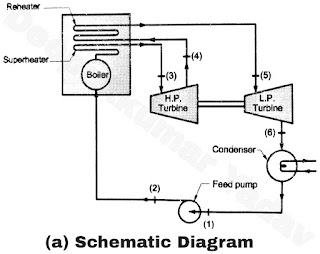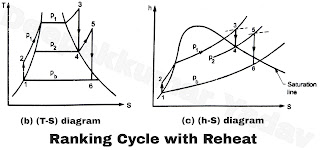# Rankine Cycle with Reheat

Rankine Cycle with ReheatFigure AFigure B
• One way of improving the efficiency of the Rankine cycle is by reheating the steam in between the stages of expansion as shown in schematic, (T-S) and (h-S) diagrams in Figure (a), (b) and (c) respectively.
• In this cycle, the steam is extracted after expansion in high pressure (H.P.) turbine at a suitable point and this steam is reheated in a reheater at constant pressure usually to its original temperature so that TT5 = T3.
• The reheating of steam in the reheater is either done with the help of flue gases from the boiler furnace or in a separate superheater by other sources of energy like electric heating.
Advantages of reheating the steam are :
1. It improves the condition of steam at exhaust of the low pressure (L.P.) turbine so that the tendency of blade erosion caused by the liquid particles in the L.P. turbine is reduced. The desirable maximum moisture in steam is considered to be about 10 to 12 percent.
2. It improves the thermal efficiency the plant since the additional heat is supplied at higher mean temperature.
3. It increases the network output of the turbine.
4. It reduces the steam rate per kWh.
Disadvantages of reheat cycle :
1. To increase the cost and size of plant due to inclusion of reheater and its long piping.
2. Increases the size of condenser based on unit mass flow of steam due to improved quality of steam at exhaust from L.P. turbine.
Analysis of cycle :
The efficiency of reheat cycle for two stage turbine with one reheater in between the stage can be computed as follows :

WT = (WT)H.P. + (WT)L.P. or  WT = (h3-h4) + (h5-h6)

WP = +(h2-h1)

Net work output, WS = WT-WP = (h3-h4) + (h5-h6)-(h2-h1)

Net heat supplied, qi = Heat supplied (in boiler + in reheater)

Or,  qi = (h3-h2) + (h5-h4)

Therefore, the thermal efficiency of the reheat cycle is given by,

η = WS/qi = (h3-h4) + (h5-h6) – (h2-h1) / (h3-h2) + (h5-h4)